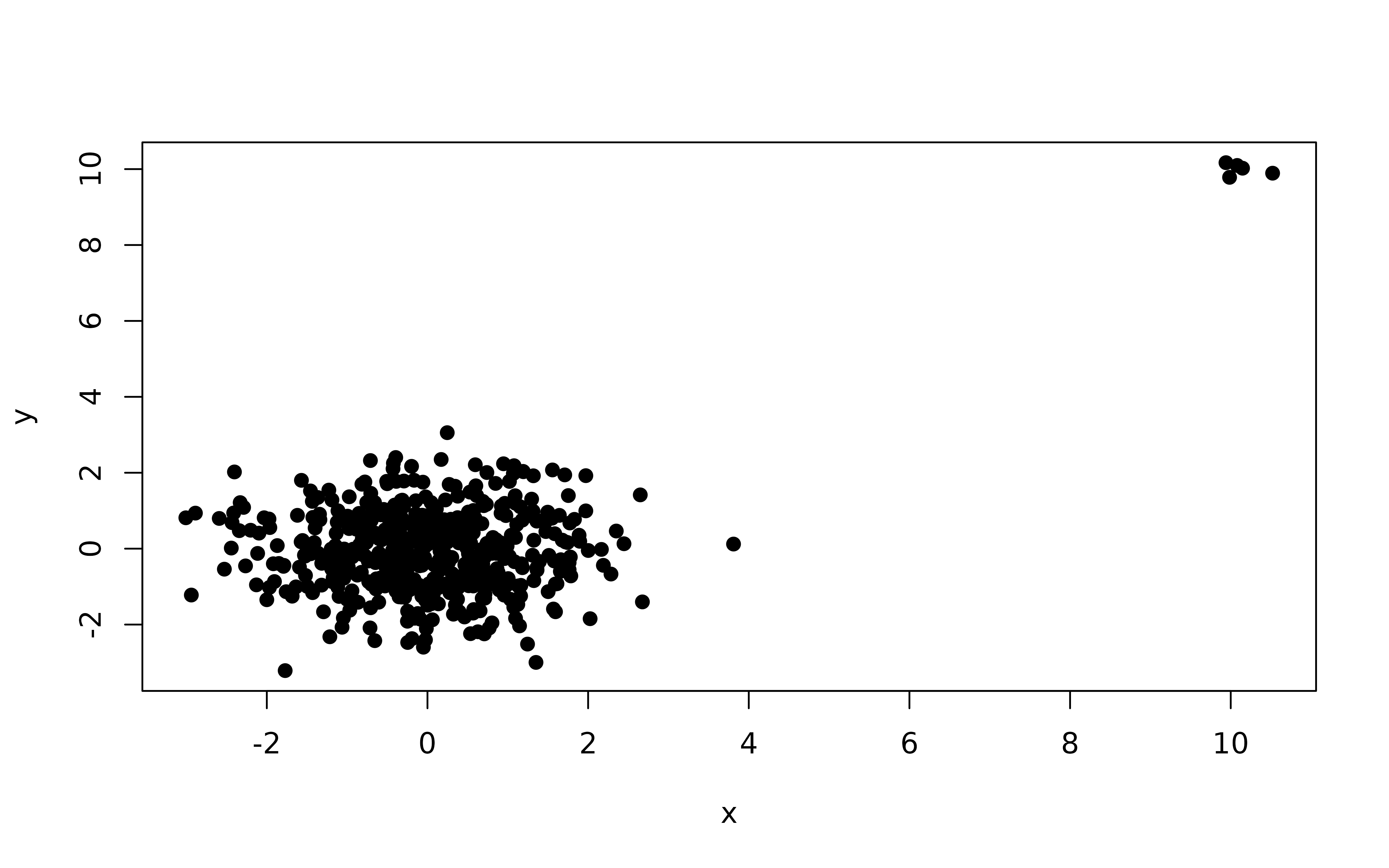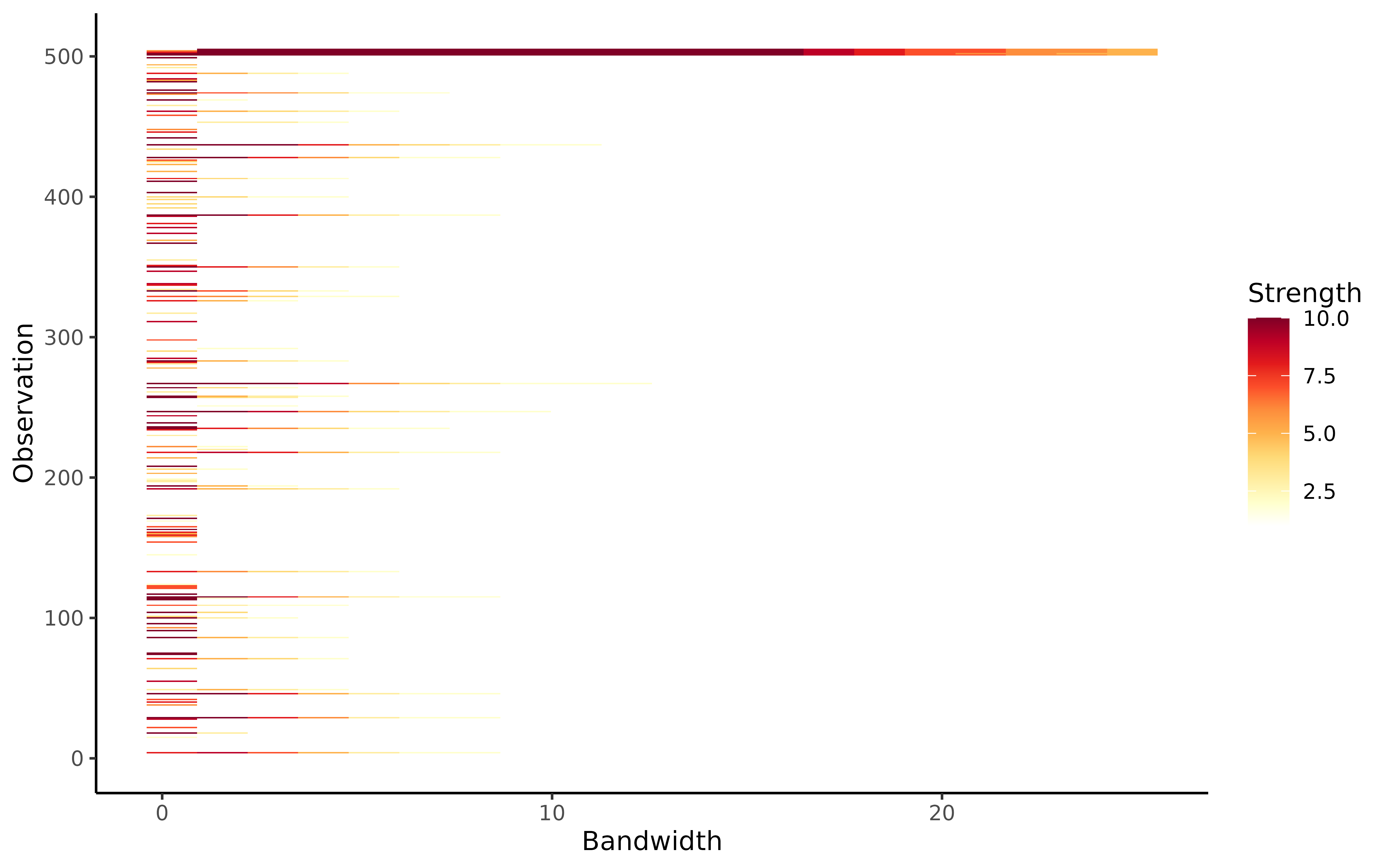This function computes outlier persistence for a range of significance values, using the algorithm lookout, an outlier detection method that uses leave-one-out kernel density estimates and generalized Pareto distributions to find outliers.

persisting_outliers(
X,
alpha = seq(0.01, 0.1, by = 0.01),
st_qq = 0.9,
unitize = TRUE,
num_steps = 20
)

## Arguments

X The input data in a matrix, data.frame, or tibble format. All columns should be numeric. Grid of significance levels. The starting quantile for death radii sequence. This will be used to compute the starting bandwidth value. An option to normalize the data. Default is TRUE, which normalizes each column to [0,1]. The length of the bandwidth sequence.

## Value

A list with the following components:

out

A 3D array of N x num_steps x num_alpha where N denotes the number of observations, num_steps denote the length of the bandwidth sequence and num_alpha denotes the number of significance levels. This is a binary array and the entries are set to 1 if that observation is an outlier for that particular bandwidth and significance level.

bw

The set of bandwidth values.

gpdparas

The GPD parameters used.

lookoutbw

The bandwidth chosen by the algorithm lookout using persistent homology.

## Examples

X <- rbind(
data.frame(x = rnorm(500),
y = rnorm(500)),
data.frame(x = rnorm(5, mean = 10, sd = 0.2),
y = rnorm(5, mean = 10, sd = 0.2))
)
plot(X, pch = 19)outliers <- persisting_outliers(X, unitize = FALSE)
outliers
#> Persistent outliers using lookout algorithm
#>
#> Call: persisting_outliers(X = X, unitize = FALSE)
#>
#> Lookout bandwidth:  2.385456 autoplot(outliers)